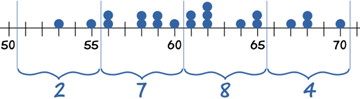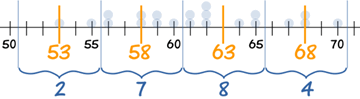# How to Determine the Mean, Median and Modefrom Grouped Frequencies

To better explain how to determine the mean, median and mode of grouped frequency data, we will work with common, relatable examples as you can see below-

Ade timed 21 people in the sprint race, to the nearest second:

59, 65, 61, 62, 53, 55, 60, 70, 64, 56, 58, 58, 62, 62, 68, 65, 56, 59, 68, 61, 67

To find the Mean, Ade adds up all the numbers, then divides by how many numbers:

 Mean = 59+65+61+62+53+55+60+70+64+56+58+58+62+62+68+65+56+59+68+61+67 21 = 61.38095…

To find the Median Ade places the numbers in value order and finds the middle number.In this case the median is the 11th number:

53, 55, 56, 56, 58, 58, 59, 59, 60, 61, 61, 62, 62, 62, 64, 65, 65, 67, 68, 68, 70

Median = 61

To find the Mode, or modal value, Ade places the numbers in value order then counts how many of each number. The Mode is the number which appears most often (there can be more than one mode):

53, 55, 56, 56, 58, 58, 59, 59, 60, 61, 61, 62, 62, 62, 64, 65, 65, 67, 68, 68, 70

62 appears three times, more often than the other values, so Mode = 62

# Grouped Frequency Table

Alex then makes a Grouped Frequency Table:

Seconds Frequency
51 – 55 2
56 – 60 7
61 – 65 8
66 – 70 4So 2 runners took between 51 and 55 seconds, 7 took between 56 and 60 seconds, etc

 Suddenly all the original data gets lost (naughty pup!) Only the Grouped Frequency Table survived …

… can we help Alex calculate the Mean, Median and Mode from just that table?

The answer is … no we can’t. Not accurately anyway. But, we can make estimates.

## Estimating the Mean from Grouped Data

So all we have left is:

Seconds Frequency
51 – 55 2
56 – 60 7
61 – 65 8
66 – 70 4

• The groups (51-55, 56-60, etc), also called class intervals, are of width 5
• The midpoints are in the middle of each class: 53, 58, 63 and 68We can estimate the Mean by using the midpoints.

So, how does this work?

Think about the 7 runners in the group 56 – 60: all we know is that they ran somewhere between 56 and 60 seconds:

• Maybe all seven of them did 56 seconds,
• Maybe all seven of them did 60 seconds,
• But it is more likely that there is a spread of numbers: some at 56, some at 57, etc

So we take an average and assume that all seven of them took 58 seconds.

Let’s now make the table using midpoints:

Midpoint Frequency
53 2
58 7
63 8
68 4Our thinking is: “2 people took 53 sec, 7 people took 58 sec, 8 people took 63 sec and 3 took 68 sec”. In other words we imagine the data looks like this:

53, 53, 58, 58, 58, 58, 58, 58, 58, 63, 63, 63, 63, 63, 63, 63, 63, 68, 68, 68, 68

Then we add them all up and divide by 21. The quick way to do it is to multiply each midpoint by each frequency:

Midpoint
x
Frequency
f
Midpoint × Frequency
fx
53 2 106
58 7 406
63 8 504
68 4 272
Totals: 21 1288

And then our estimate of the mean time to complete the race is:

 Estimated Mean = 1288 = 61.333… 21

Very close to the exact answer we got earlier.